GATE  >  Practice Test: Gate Civil Engineering(CE) 2016 Paper

# Practice Test: Gate Civil Engineering(CE) 2016 Paper - GATE

Test Description

## 65 Questions MCQ Test - Practice Test: Gate Civil Engineering(CE) 2016 Paper

Practice Test: Gate Civil Engineering(CE) 2016 Paper for GATE 2023 is part of GATE preparation. The Practice Test: Gate Civil Engineering(CE) 2016 Paper questions and answers have been prepared according to the GATE exam syllabus.The Practice Test: Gate Civil Engineering(CE) 2016 Paper MCQs are made for GATE 2023 Exam. Find important definitions, questions, notes, meanings, examples, exercises, MCQs and online tests for Practice Test: Gate Civil Engineering(CE) 2016 Paper below.
Solutions of Practice Test: Gate Civil Engineering(CE) 2016 Paper questions in English are available as part of our course for GATE & Practice Test: Gate Civil Engineering(CE) 2016 Paper solutions in Hindi for GATE course. Download more important topics, notes, lectures and mock test series for GATE Exam by signing up for free. Attempt Practice Test: Gate Civil Engineering(CE) 2016 Paper | 65 questions in 180 minutes | Mock test for GATE preparation | Free important questions MCQ to study for GATE Exam | Download free PDF with solutions
 1 Crore+ students have signed up on EduRev. Have you?
Practice Test: Gate Civil Engineering(CE) 2016 Paper - Question 1

### Q. 1 – Q. 5 carry one mark each. Out of the following four sentences, select the most suitable sentence with respect to grammar and usage.

Detailed Solution for Practice Test: Gate Civil Engineering(CE) 2016 Paper - Question 1

‘until’ itself is negative so it can’t take one more negative i.e., ‘does not’. Hence, Option (D) is the right answer

Practice Test: Gate Civil Engineering(CE) 2016 Paper - Question 2

### A rewording of something written or spoken is a ______________.

Detailed Solution for Practice Test: Gate Civil Engineering(CE) 2016 Paper - Question 2

‘paraphrase’ means a restatement of a text, passage or a rewording of something written or spoken.

Practice Test: Gate Civil Engineering(CE) 2016 Paper - Question 3

### Archimedes said, “Give me a lever long enough and a fulcrum on which to place it, and I will move the world.” The sentence above is an example of a ___________ statement

Detailed Solution for Practice Test: Gate Civil Engineering(CE) 2016 Paper - Question 3

‘figurative’ means representing by a figure or resemblance or expressing one thing in terms
normally denoting another with which it may be regarded as analogous.

Practice Test: Gate Civil Engineering(CE) 2016 Paper - Question 4

A cube is built using 64 cubic blocks of side one unit. After it is built, one cubic block is removed from every corner of the cube. The resulting surface area of the body (in square units) after the removal is __________.

Detailed Solution for Practice Test: Gate Civil Engineering(CE) 2016 Paper - Question 4

From given data, 64 cubic blocks of one unit
Sizes are formed
No of faces of the Cube is ‘6’
No of corners of the Cube is ‘8’
After removing one Cubic block from
Each corner, The resulting surface area of the body = 6 * (4) = 96 sq. Units.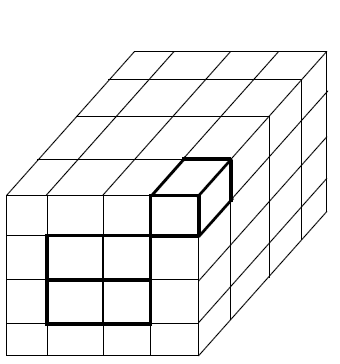Practice Test: Gate Civil Engineering(CE) 2016 Paper - Question 5

If ‘relftaga’ means carefree, ‘otaga’ means careful and ‘fertaga’ means careless, which of the following could mean ‘aftercare’?

Detailed Solution for Practice Test: Gate Civil Engineering(CE) 2016 Paper - Question 5

‘taga’ and ‘care’ are a matching pair in every combination.
So, ‘taga’ surely represents ‘care’.

Also, note here that the second half of the word in encoded value refers to the first half of the word in the decoded value.
So, ‘fer’ represents ‘less’, ‘relf’ represents ‘free’ and ‘o’ represents ‘full’.

Going by the same logic, the answer would be tagazen, i.e., C.

Practice Test: Gate Civil Engineering(CE) 2016 Paper - Question 6

Q. 6 – Q. 10 carry two marks each.

A shaving set company sells 4 different types of razors, Elegance, Smooth, Soft and Executive. Elegance sells at Rs. 48, Smooth at Rs. 63, Soft at Rs. 78 and Executive at Rs. 173 per piece. The table below shows the numbers of each razor sold in each quarter of a year.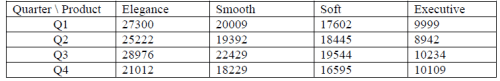Which product contributes the greatest fraction to the revenue of the company in that year?

Detailed Solution for Practice Test: Gate Civil Engineering(CE) 2016 Paper - Question 6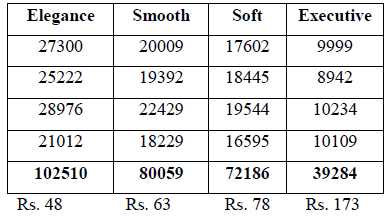Total Revenue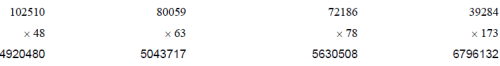More revenue is on executive

Practice Test: Gate Civil Engineering(CE) 2016 Paper - Question 7

Indian currency notes show the denomination indicated in at least seventeen languages. If this is not an indication of the nation’s diversity, nothing else is.

Which of the following can be logically inferred from the above sentences?

Detailed Solution for Practice Test: Gate Civil Engineering(CE) 2016 Paper - Question 7

If seventeen languages were not an indication of the nation’s diversity, nothing else is. If nothing else is so the best inference is option ‘D’

Practice Test: Gate Civil Engineering(CE) 2016 Paper - Question 8

Consider the following statements relating to the level of poker play of four players P, Q, R and S.

I.    P always beats Q
II.   R always beats S
III.  S loses to P only sometimes
IV.  R always loses to Q

Which of the following can be logically inferred from the above statements?

(i) P is likely to beat all the three other players
(ii) S is the absolute worst player in the set

Detailed Solution for Practice Test: Gate Civil Engineering(CE) 2016 Paper - Question 8

From the given data
Player P > Player Q
Player R > Player S
Player S < Player P (sometimes only)
Player R < Player Q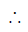Player ‘P’ > Player ‘Q’ > Player ‘R’ > Player ‘S’Logically, the statement (i) is definitely true, but statement (ii) is notOption ‘A’ is true

Practice Test: Gate Civil Engineering(CE) 2016 Paper - Question 9

If f(x) = 2x7+3x−5, which of the following is a factor of f(x)?

Detailed Solution for Practice Test: Gate Civil Engineering(CE) 2016 Paper - Question 9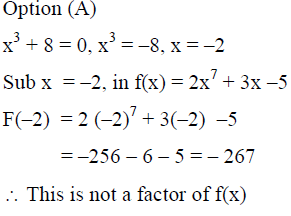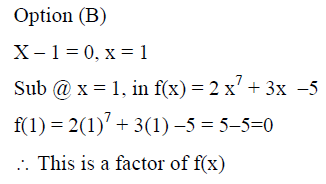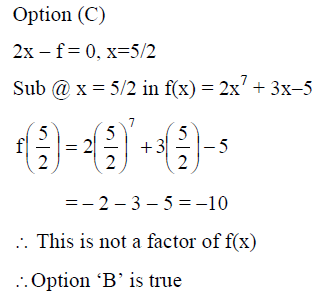Practice Test: Gate Civil Engineering(CE) 2016 Paper - Question 10

In a process, the number of cycles to failure decreases exponentially with an increase in load. At a load of 80 units, it takes 100 cycles for failure. When the load is halved, it takes 10000 cycles for failure. The load for which the failure will happen in 5000 cycles is ________.

Detailed Solution for Practice Test: Gate Civil Engineering(CE) 2016 Paper - Question 10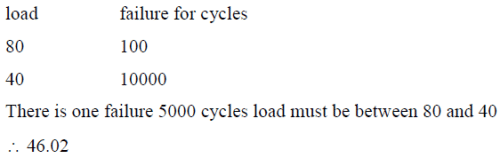Practice Test: Gate Civil Engineering(CE) 2016 Paper - Question 11

The number of simultaneous equations to be solved in the slope deflection method, is equal to :

Detailed Solution for Practice Test: Gate Civil Engineering(CE) 2016 Paper - Question 11

Slope Deflection Method, is a type of displacement method. In displacement method, the deflection and rotation are unknowns. For every joint we can write a relation between the moments and displacements. Thus, to solve for the structure number of simultaneous equations to be solved in the slope deflection method is equal to the number of joints in the structure.

Practice Test: Gate Civil Engineering(CE) 2016 Paper - Question 12

The type of partial differential equation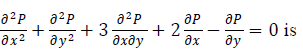Detailed Solution for Practice Test: Gate Civil Engineering(CE) 2016 Paper - Question 12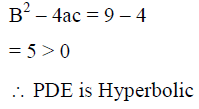Practice Test: Gate Civil Engineering(CE) 2016 Paper - Question 13

If the entries in each column of a square matrix M add up to 1, then an eigenvalue of M is

Detailed Solution for Practice Test: Gate Civil Engineering(CE) 2016 Paper - Question 13

All Options are correct

Practice Test: Gate Civil Engineering(CE) 2016 Paper - Question 14

Type II error in hypothesis testing is

Detailed Solution for Practice Test: Gate Civil Engineering(CE) 2016 Paper - Question 14

Type II error means acceptance of the null hypothesis when it is false and should be
rejected

Practice Test: Gate Civil Engineering(CE) 2016 Paper - Question 15

The number of revolutions of a current meter in 50 seconds were found to be 12 and 30 corresponding to the velocities of 0.25 and 0.46 m/s respectively. What velocity (in m/s) would be indicated by 50 revolutions of that current meter in one minute ?

Detailed Solution for Practice Test: Gate Civil Engineering(CE) 2016 Paper - Question 15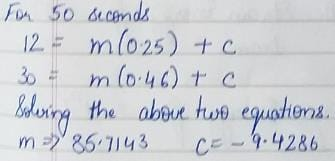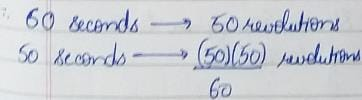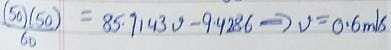Practice Test: Gate Civil Engineering(CE) 2016 Paper - Question 16

Consider the plane truss with load P as shown in the figure. Let the horizontal and vertical reactions at the joint B be HB and VB, respectively and VC be the vertical reaction at the joint C.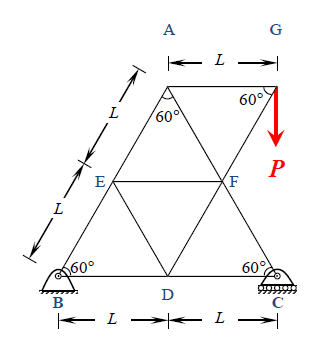Which one of the following sets gives the correct values of VB, HB and VC?

Detailed Solution for Practice Test: Gate Civil Engineering(CE) 2016 Paper - Question 16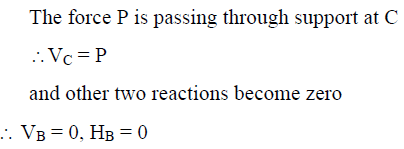Practice Test: Gate Civil Engineering(CE) 2016 Paper - Question 17

In shear design of an RC beam, other than the allowable shear strength of concrete ( τc), there is also an additional check suggested in IS 456-2000 with respect to the maximum permissible shear stress ( τcmax) . The check for τcmax is required to take care of

Detailed Solution for Practice Test: Gate Civil Engineering(CE) 2016 Paper - Question 17

If Tv > Tc max , diagonal compression failure occurs in concrete

*Answer can only contain numeric values
Practice Test: Gate Civil Engineering(CE) 2016 Paper - Question 18

The semi-compact section of a laterally unsupported steel beam has an elastic section modulus, plastic section modulus and design bending compressive stress of 500 cm3, 650 cm3 and 200 MPa, respectively. The design flexural capacity (expressed in kNm) of the section is _________

Detailed Solution for Practice Test: Gate Civil Engineering(CE) 2016 Paper - Question 18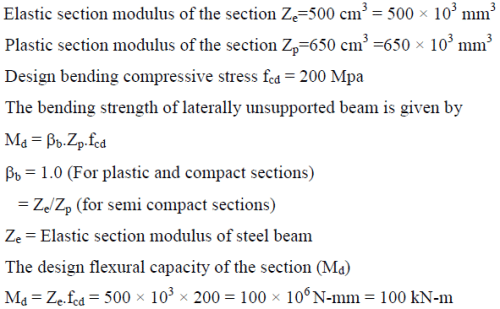Practice Test: Gate Civil Engineering(CE) 2016 Paper - Question 19

Bull's trench kiln is used in the manufacturing of

Detailed Solution for Practice Test: Gate Civil Engineering(CE) 2016 Paper - Question 19

Bulls Trench kiln is used for manufacturing bricks

Practice Test: Gate Civil Engineering(CE) 2016 Paper - Question 20

The compound which is largely responsible for initial setting and early strength gain of Ordinary Portland Cement is

Detailed Solution for Practice Test: Gate Civil Engineering(CE) 2016 Paper - Question 20

The compound responsible for initial setting and early strength gain in OPC is C3S

Practice Test: Gate Civil Engineering(CE) 2016 Paper - Question 21

In the consolidated undrained trixal test on a saturated soil sample, the pore water pressure is zero

Detailed Solution for Practice Test: Gate Civil Engineering(CE) 2016 Paper - Question 21

In consolidated undrained test, at the first stage consolidation will be allowed to take place. Since, consolidation takes place due to excess pore water pressure, the soil will continue to consolidate and at the end of consolidation stage will be completed implying pore water pressure in the soil to be zero.
Since, in the second stage drainage is not allowed, thus, water may drain in or out during the shearing stage thus, leading to development of pore water pressure again.

Practice Test: Gate Civil Engineering(CE) 2016 Paper - Question 22

A fine grained soil is found to be plastic in the water content range of 26-48%. As per Indian standard classificatin system, the soil is classified as

Detailed Solution for Practice Test: Gate Civil Engineering(CE) 2016 Paper - Question 22

wP = 26%,
wL = 48%
Since wL lies in the range of 35 - 50%, it is intermediate compressible. Hence soils is CI.

Practice Test: Gate Civil Engineering(CE) 2016 Paper - Question 23

A vertical cut is to be made in a soil mass having cohesion c, angle of internal friction φ, and unit wieght γ, considering kand kp as the coefficients of active and passive earth pressure, respectively, the maximum depth of unsupported excavation is

Detailed Solution for Practice Test: Gate Civil Engineering(CE) 2016 Paper - Question 23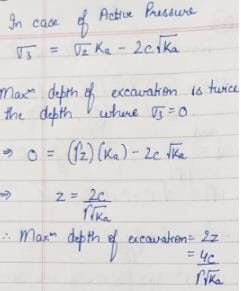*Answer can only contain numeric values
Practice Test: Gate Civil Engineering(CE) 2016 Paper - Question 24

The direct runoff hydrograph in response to 5cm rainfall excess in a catchment is shown in the figure. The area of the catchment (expressed in hectares) is ____________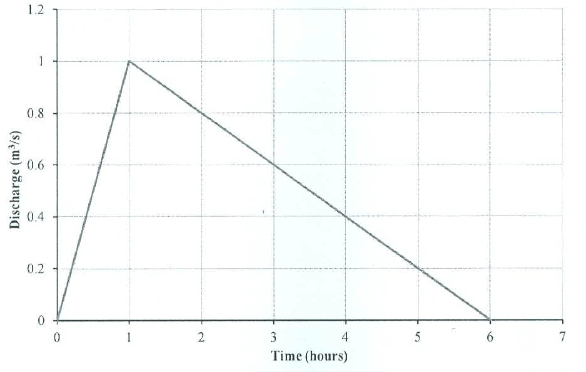Detailed Solution for Practice Test: Gate Civil Engineering(CE) 2016 Paper - Question 24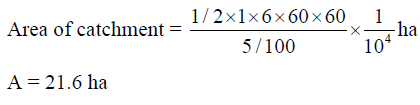Practice Test: Gate Civil Engineering(CE) 2016 Paper - Question 25

The type of flood routing (Group I) and the equation(s) used for the purpose (Group II) are given below.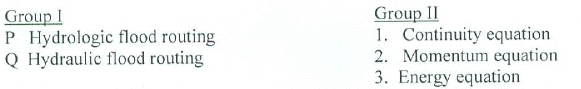The correct match is

Detailed Solution for Practice Test: Gate Civil Engineering(CE) 2016 Paper - Question 25

Hydrologic Routing-Also known as lumped routing. Flow is only a function of time. Considers the use of continuity equation,
Hydraulic Flood Routing-Also known as distributed routing. Flow is a function of space and time. Considers the use of continuity equation and momentum equation.

*Answer can only contain numeric values
Practice Test: Gate Civil Engineering(CE) 2016 Paper - Question 26

The pre-jump Froude number for a particular flow in a horizontal rectangular channel is 10. The ratio of sequent depths (i.e., post-jump depth to pre-jump depth) is ____________

Detailed Solution for Practice Test: Gate Civil Engineering(CE) 2016 Paper - Question 26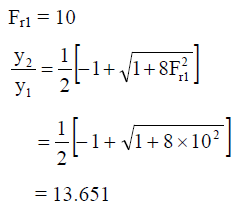Practice Test: Gate Civil Engineering(CE) 2016 Paper - Question 27

Pre-cursors to photochemical oxidants are

Practice Test: Gate Civil Engineering(CE) 2016 Paper - Question 28

Crown corrosion in a reinforced concrete sewer is caused by:

Practice Test: Gate Civil Engineering(CE) 2016 Paper - Question 29

It was decided to construct a fabric filter, using bags of 0.45 m diameter and 7.5 m long, for removing industrial stack gas containing particulates. The expected rate of airflow into the filter is 10 m3/s. If the filtering velocity is 2.0 m/min, the minimum number of bags (rounded to nearest higher integer) required for continuous cleaning operation is

Detailed Solution for Practice Test: Gate Civil Engineering(CE) 2016 Paper - Question 29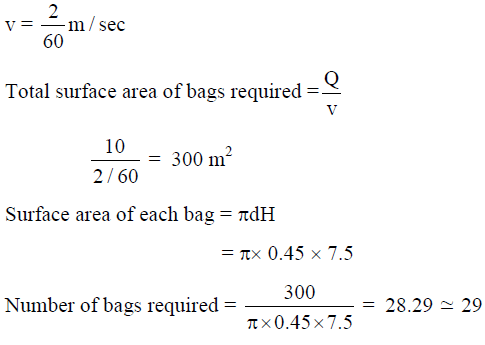Practice Test: Gate Civil Engineering(CE) 2016 Paper - Question 30

Match the items in Group – I with those in Group – II and choose the right combination.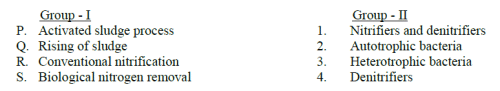Practice Test: Gate Civil Engineering(CE) 2016 Paper - Question 31

During a forensic investigation of pavement failure, an engineer reconstructed the graphs P, Q, R and S, using partial and damaged old reports.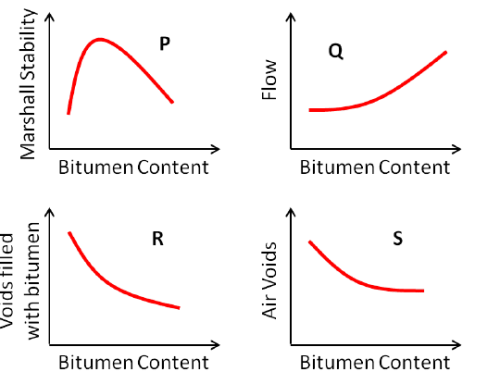Theoretically plausible correct graphs according to the 'Marshall mixture design output' are

Detailed Solution for Practice Test: Gate Civil Engineering(CE) 2016 Paper - Question 31

The graph wrong among the given is ‘R’
The correct graph should be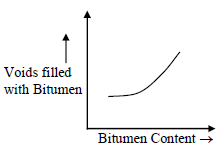*Answer can only contain numeric values
Practice Test: Gate Civil Engineering(CE) 2016 Paper - Question 32

In a one-lane one-way homogeneous traffic stream, the observed average headway is 3.0 s. The flow (expressed in vehicles/hr) in this traffic stream is________

Detailed Solution for Practice Test: Gate Civil Engineering(CE) 2016 Paper - Question 32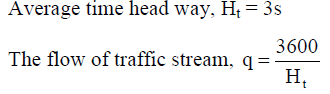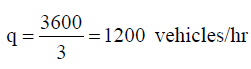Practice Test: Gate Civil Engineering(CE) 2016 Paper - Question 33

Some of the structural strength of a clayey material that is lost by remoulding is slowly recovered with time. This property of soils to undergo an isothermal gel-to-soil-to-gel transformation upon agitation and subsequent rest is termed :

Practice Test: Gate Civil Engineering(CE) 2016 Paper - Question 34

The system that uses the Sun as a source of electromagnetic energy and records the naturally radiated and reflected energy from the object is called

Detailed Solution for Practice Test: Gate Civil Engineering(CE) 2016 Paper - Question 34

Geographical Information System-A geographic information system (GIS) is a system designed to capture, store, manipulate, analyze, manage, and present all types of geographical data.
Global Positioning System- Developed by USA, uses satellites to position/locate a point on map.
Passive Remote Sensing- The system that uses the Sun as a source of electromagnetic energy and records the naturally radiated and reflected energy from the object.
Active Remote Sensing- Active sensors, provide their own source of energy to illuminate the objects they observe.

Practice Test: Gate Civil Engineering(CE) 2016 Paper - Question 35

The staff reading taken on a workshop floor using a level is 0.645 m. The inverted staff reading taken to the bottom of a beam is 2.960 m. The reduced level of the floor is 40.500 m. The reduced level (expressed in m) of the bottom of the beam is

Detailed Solution for Practice Test: Gate Civil Engineering(CE) 2016 Paper - Question 35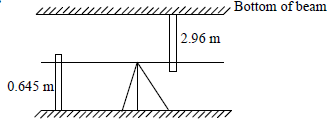Floor reduced level = 40.5 m
RL of bottom of beam = 40.5 + 0.645 + 2.96
= 44.105 m

Practice Test: Gate Civil Engineering(CE) 2016 Paper - Question 36

Q. 36 – Q. 65 carry two marks each

Probability density function of a random variable X is given below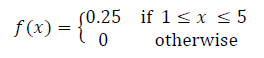P(X ≤ 4) is

Detailed Solution for Practice Test: Gate Civil Engineering(CE) 2016 Paper - Question 36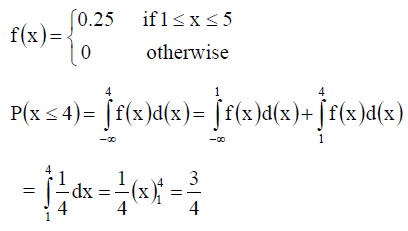Practice Test: Gate Civil Engineering(CE) 2016 Paper - Question 37

The value of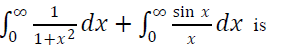Detailed Solution for Practice Test: Gate Civil Engineering(CE) 2016 Paper - Question 37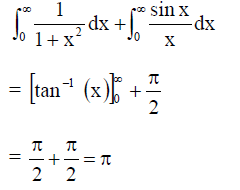Practice Test: Gate Civil Engineering(CE) 2016 Paper - Question 38

The area of the region bounded by the parabola y= x2+ 1 and the straight line x+y=3 is

Detailed Solution for Practice Test: Gate Civil Engineering(CE) 2016 Paper - Question 38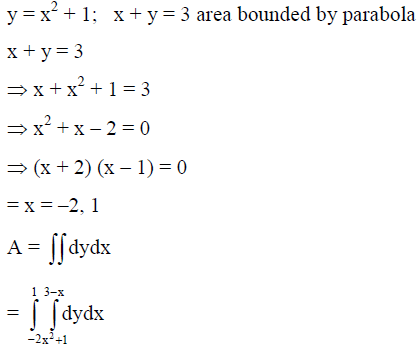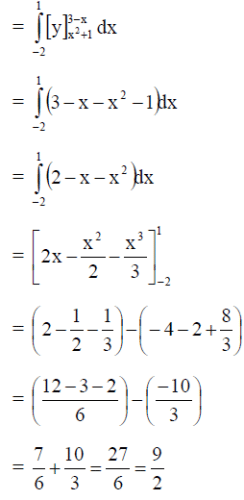Practice Test: Gate Civil Engineering(CE) 2016 Paper - Question 39

The magnitudes of vectors P, Q and R are 100 kN, 250 kN and 150 kN, respectively as shown in the figure.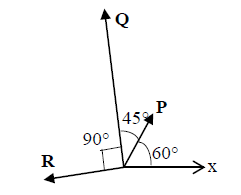The respective values of the magnitude (in kN) and the direction (with respect to the x-axis) of the resultant vector are

Detailed Solution for Practice Test: Gate Civil Engineering(CE) 2016 Paper - Question 39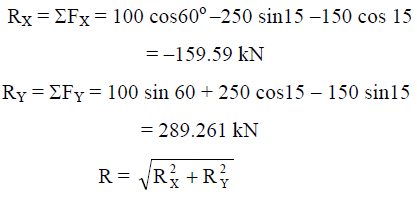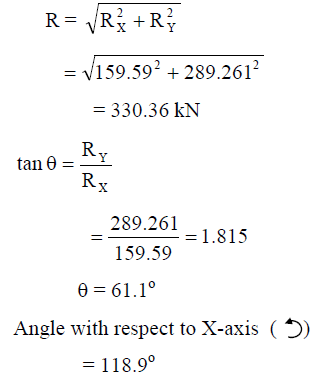Practice Test: Gate Civil Engineering(CE) 2016 Paper - Question 40

At a rated capacity of 44 Cumecs, a centrifugal pump develops 36 m of head when operating at 1450 rpm. Its specific speed is :

Detailed Solution for Practice Test: Gate Civil Engineering(CE) 2016 Paper - Question 40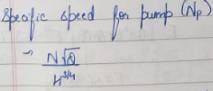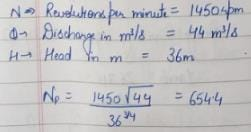Practice Test: Gate Civil Engineering(CE) 2016 Paper - Question 41

A 3 m long simply supported beam of uniform cross section is subjected to a uniformly distributed load of w = 20 kN/m in the central 1 m as shown in the figure.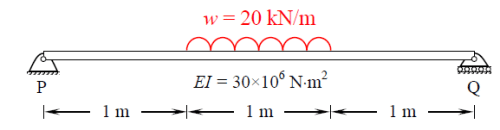If the flexural rigidity (EI) of the beam is 30 x 106 N-m2, the maximum slope (expressed in radians) of the deformed beam is

Detailed Solution for Practice Test: Gate Civil Engineering(CE) 2016 Paper - Question 41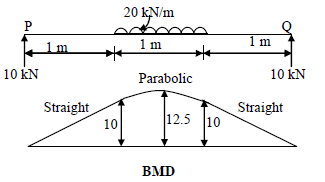Max. BM at the centre of beam = 10*1.5 – 20*0.5*0.25 = 12.5 kN-m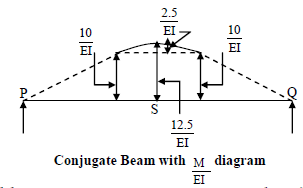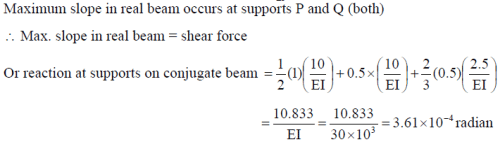Practice Test: Gate Civil Engineering(CE) 2016 Paper - Question 42

Two beams PQ (fixed at P and with a roller support at Q, as shown in Figure I, which allows vertical movement) and XZ (with a hinge at Y) are shown in the Figures I and II respectively. The spans of PQ and XZ are L and 2L respectively. Both the beams are under the action of uniformly distributed load (W) and have the same flexural stiffness, EI (where, E and I respectively denote modulus of elasticity and moment of inertia about axis of bending). Let the maximum deflection and maximum rotation be δmax1 and θmax1, respectively, in the case of beam PQ and the corresponding quantities for the beam XZ be δmax2 and θmax2, respectively.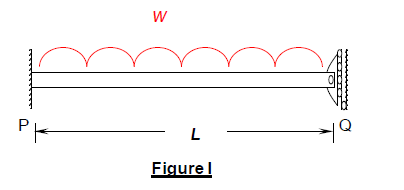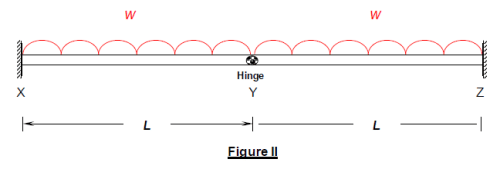Which one of the following relationships is true?

Detailed Solution for Practice Test: Gate Civil Engineering(CE) 2016 Paper - Question 42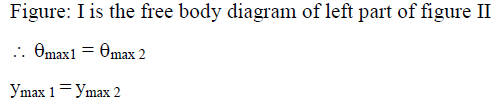Practice Test: Gate Civil Engineering(CE) 2016 Paper - Question 43

A plane truss with applied loads is shown in the figure.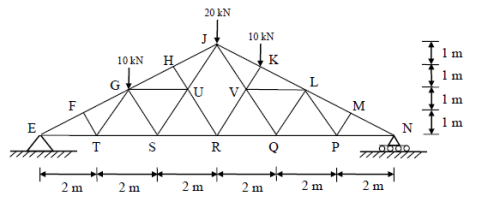The members which do not carry any force are

Detailed Solution for Practice Test: Gate Civil Engineering(CE) 2016 Paper - Question 43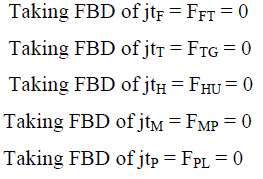Practice Test: Gate Civil Engineering(CE) 2016 Paper - Question 44

A rigid member ACB is shown in the figure. The member is supported at A and B by pinned and guided roller supports, respectively. A force P acts at C as shown. Let RAh and RBh be the horizontal reactions at supports A and B, respectively, and RAv be the vertical reaction at support A. Selfweight of the member may be ignored.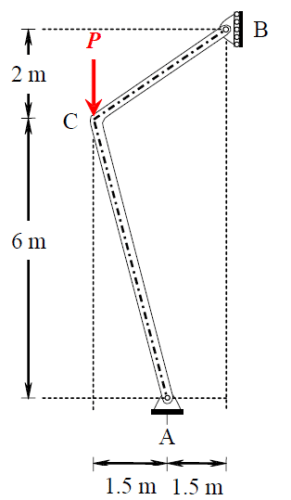Which one of the following sets gives the correct magnitudes of RAv, RBh and RAh ?

Detailed Solution for Practice Test: Gate Civil Engineering(CE) 2016 Paper - Question 44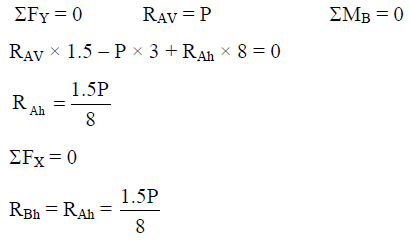Practice Test: Gate Civil Engineering(CE) 2016 Paper - Question 45

A reinforced concrete (RC) beam with width of 250 mm and effective depth of 400 mm is reinforced with Fe415 steel. As per the provisions of IS 456-2000, the minimum and maximum amount of tensile reinforcement (expressed in mm2) for the section are, respectively

Detailed Solution for Practice Test: Gate Civil Engineering(CE) 2016 Paper - Question 45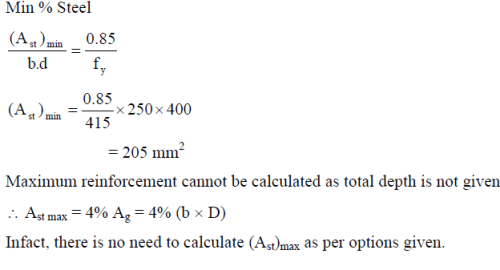*Answer can only contain numeric values
Practice Test: Gate Civil Engineering(CE) 2016 Paper - Question 46

For M25 concrete with creep coefficient of 1.5, the long-term static modulus of elasticity (expressed in MPa) as per the provisions of IS:456-2000 is ________

Detailed Solution for Practice Test: Gate Civil Engineering(CE) 2016 Paper - Question 46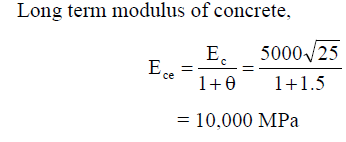Practice Test: Gate Civil Engineering(CE) 2016 Paper - Question 47

A propped cantilever of span L carries a vertical concentrated load at the mid-span. If the plastic moment capacity of the section is Mp, the magnitude of the collapse load is

Detailed Solution for Practice Test: Gate Civil Engineering(CE) 2016 Paper - Question 47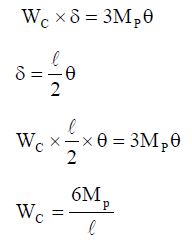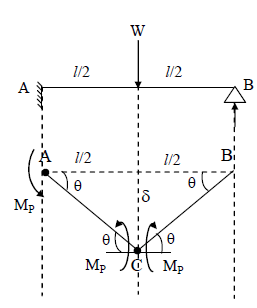Practice Test: Gate Civil Engineering(CE) 2016 Paper - Question 48

Two plates are connected by fillet welds of size 10 mm and subjected to tension, as shown in the figure. The thickness of each plate is 12 mm. The yield stress and the ultimate tensile stress of steel are 250 MPa and 410 MPa, respectively. The welding is done in the workshop (γmw = 1.25).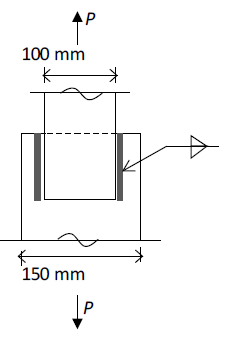As per the Limit State Method of IS 800: 2007, the minimum length (rounded off to the nearest higher multiple of 5 mm) of each weld to transmit a force P equal to 270 kN (factored) is

Detailed Solution for Practice Test: Gate Civil Engineering(CE) 2016 Paper - Question 48*Answer can only contain numeric values
Practice Test: Gate Civil Engineering(CE) 2016 Paper - Question 49

The Optimistic Time (O), Most likely Time (M) and Pessimistic Time (P) (in days) of the activities in the critical path are given below in the format O-M-P.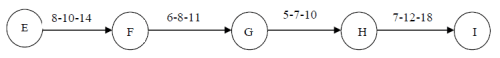The expected completion time (in days) of the project is _________

Detailed Solution for Practice Test: Gate Civil Engineering(CE) 2016 Paper - Question 49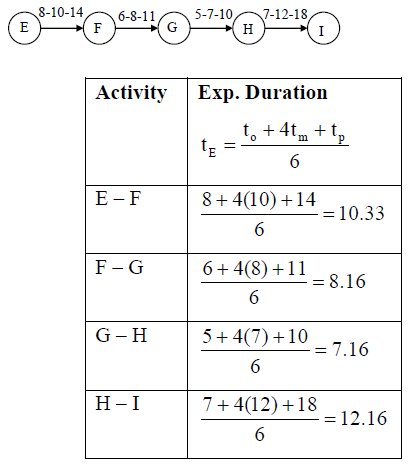Expected completion time of the project = 10.33 + 8.16 + 7.16 + 12.16
= 37.81 days

*Answer can only contain numeric values
Practice Test: Gate Civil Engineering(CE) 2016 Paper - Question 50

The porosity (n) and the degree of saturation (S) of a soil sample are 0.7 and 40%, respectively. In a 100 m3 volume of the soil, the volume (expressed in m3) of air is _________

Detailed Solution for Practice Test: Gate Civil Engineering(CE) 2016 Paper - Question 50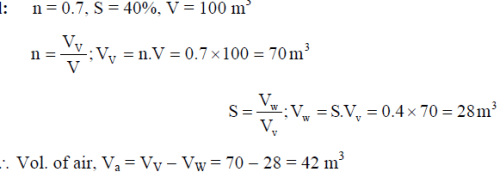Practice Test: Gate Civil Engineering(CE) 2016 Paper - Question 51

A homogeneous gravity retaining wall supporting a cohesionless backfill is shown in the figure. The lateral active earth pressure at the bottom of the wall is 40 kPa.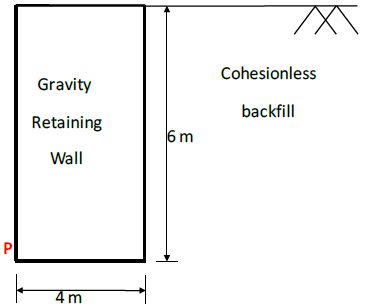The minimum weight of the wall (expressed in kN per m length) required to prevent it from overturning about its toe (Point P) is

Detailed Solution for Practice Test: Gate Civil Engineering(CE) 2016 Paper - Question 51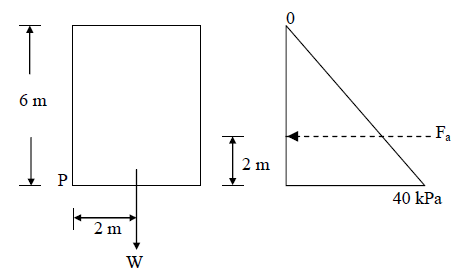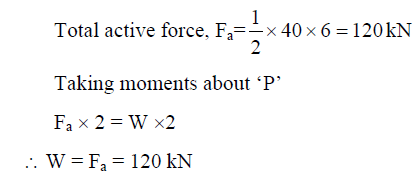*Answer can only contain numeric values
Practice Test: Gate Civil Engineering(CE) 2016 Paper - Question 52

An undisturbed soil sample was taken from the middle of a clay layer (i.e., 1.5 m below GL), as shown in figure. The water table was at the top of clay layer. Laboratory test results are as follows: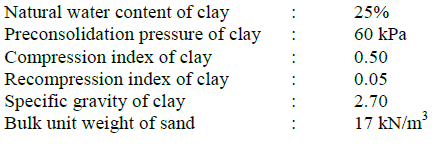A compacted fill of 2.5 m height with unit weight of 20 kN/m3 is placed at the ground level.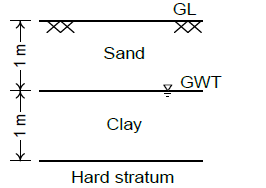Assuming unit weight of water as10 kN/m3, the ultimate consolidation settlement (expressed in mm) of the clay layer is ____________

Detailed Solution for Practice Test: Gate Civil Engineering(CE) 2016 Paper - Question 52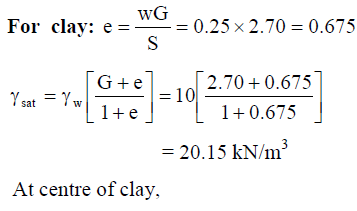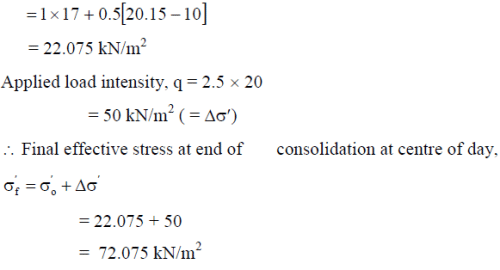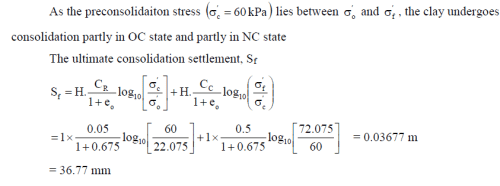*Answer can only contain numeric values
Practice Test: Gate Civil Engineering(CE) 2016 Paper - Question 53

A seepage flow condition is shown in the figure. The saturated unit weight of the soil γsat = 18 kN/m3. Using unit weight of water, γw = 9.81 kN/m3, the effective vertical stress (expressed in kN/m2) on plane X-X is ________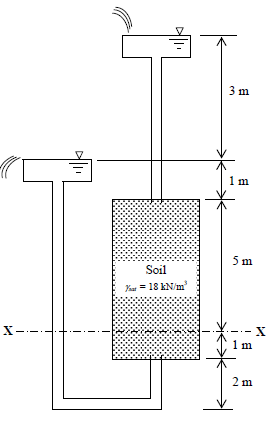Detailed Solution for Practice Test: Gate Civil Engineering(CE) 2016 Paper - Question 53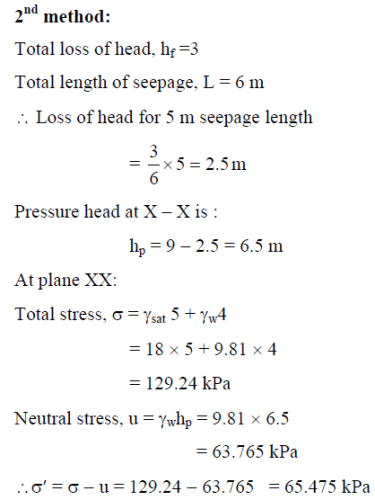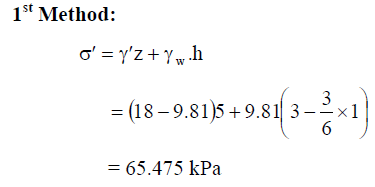*Answer can only contain numeric values
Practice Test: Gate Civil Engineering(CE) 2016 Paper - Question 54

A drained triaxial compression test on a saturated clay yielded the effective shear strength parameters as c' = 15 kPa and φ' = 22°. Consolidated Undrained triaxial test on an identical sample of this clay at a cell pressure of 200 kPa developed a pore water pressure of 150 kPa at failure. The deviator stress (expressed in kPa) at failure is _________

Detailed Solution for Practice Test: Gate Civil Engineering(CE) 2016 Paper - Question 54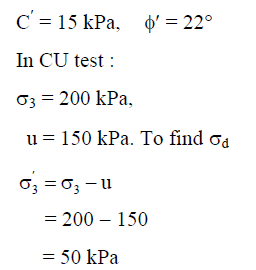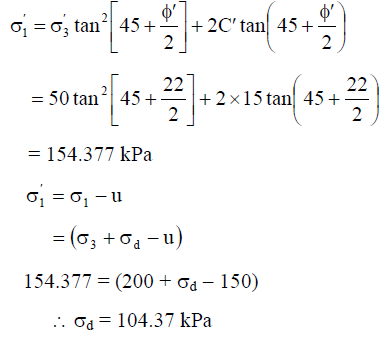*Answer can only contain numeric values
Practice Test: Gate Civil Engineering(CE) 2016 Paper - Question 55

A concrete gravity dam section is shown in the figure. Assuming unit weight of water as 10 kN/m3 and unit weight of concrete as 24 kN/m3, the uplift force per unit length of the dam (expressed in kN/m) at PQ is _________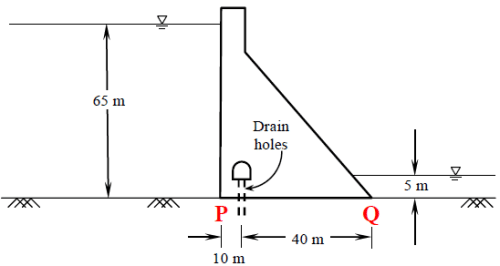Detailed Solution for Practice Test: Gate Civil Engineering(CE) 2016 Paper - Question 55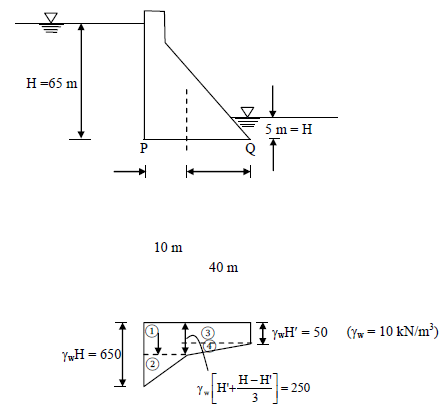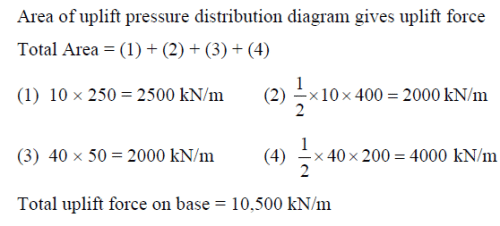Practice Test: Gate Civil Engineering(CE) 2016 Paper - Question 56

Seepage is occurring through a porous media shown in the figure. The hydraulic conductivity values (k1, k2, k3) are in m/day.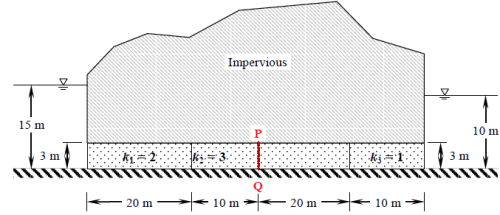The seepage discharge (m3/day per m) through the porous media at section PQ is

Detailed Solution for Practice Test: Gate Civil Engineering(CE) 2016 Paper - Question 56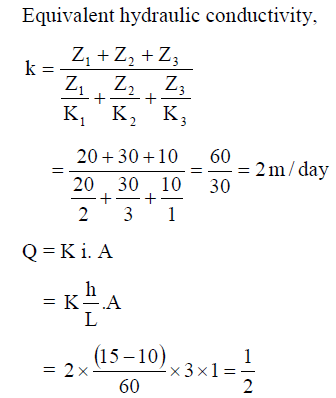Practice Test: Gate Civil Engineering(CE) 2016 Paper - Question 57

A 4 m wide rectangular channel, having bed slope of 0.001 carries a discharge of 16 m3/s. Considering Manning's roughness coefficient = 0.012 and g = 10 m/s2, the category of the channel slope is

Detailed Solution for Practice Test: Gate Civil Engineering(CE) 2016 Paper - Question 57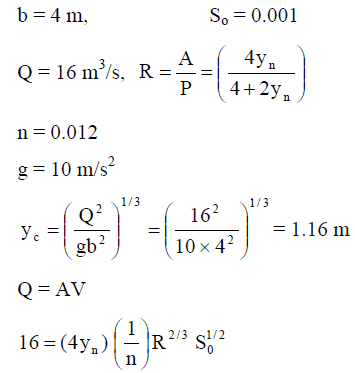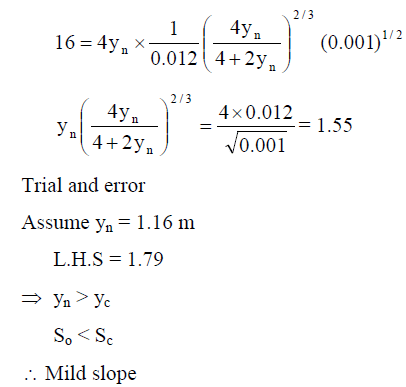*Answer can only contain numeric values
Practice Test: Gate Civil Engineering(CE) 2016 Paper - Question 58

A sector gate is provided on a spillway as shown in the figure. Assuming g = 10 m/s2, the resultant force per meter length (expressed in kN/m) on the gate will be __________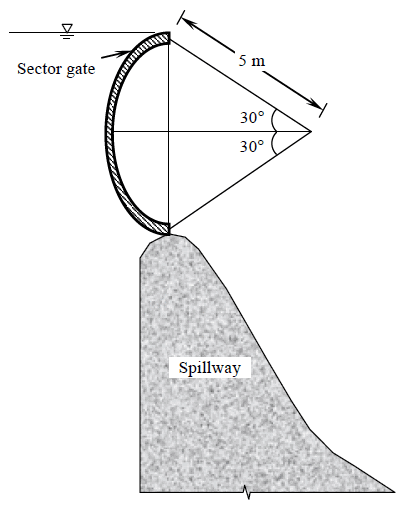Detailed Solution for Practice Test: Gate Civil Engineering(CE) 2016 Paper - Question 58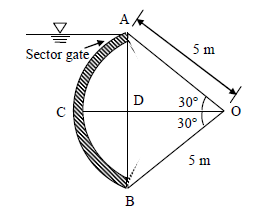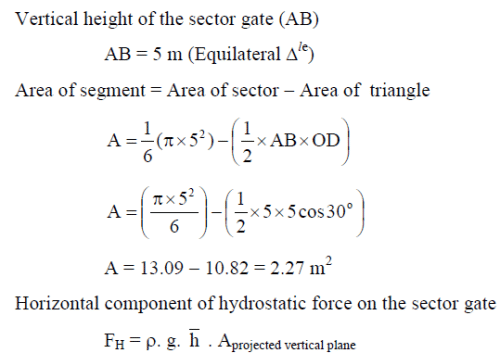*Answer can only contain numeric values
Practice Test: Gate Civil Engineering(CE) 2016 Paper - Question 59

A hydraulically efficient trapezoidal channel section has a uniform flow depth of 2 m. The bed width (expressed in m) of the channel is __________

Detailed Solution for Practice Test: Gate Civil Engineering(CE) 2016 Paper - Question 59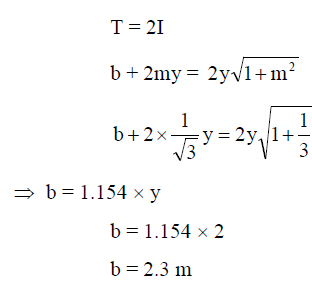*Answer can only contain numeric values
Practice Test: Gate Civil Engineering(CE) 2016 Paper - Question 60

Effluent from an industry 'A' has a pH of 4.2. The effluent from another industry 'B' has double the hydroxyl (OH-) ion concentration than the effluent from industry 'A'. pH of effluent from the industry 'B' will be __________

Detailed Solution for Practice Test: Gate Civil Engineering(CE) 2016 Paper - Question 60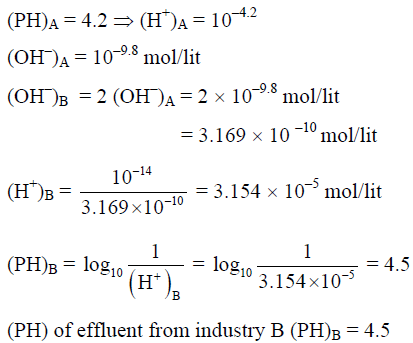*Answer can only contain numeric values
Practice Test: Gate Civil Engineering(CE) 2016 Paper - Question 61

An electrostatic precipitator (ESP) with 5600 m2 of collector plate area is 96 percent efficient in treating 185 m3/s of flue gas from a 200 MW thermal power plant. It was found that in order to achieve 97 percent efficiency, the collector plate area should be 6100 m2. In order to increase the efficiency to 99 percent, the ESP collector plate area (expressed in m2) would be ______

Detailed Solution for Practice Test: Gate Civil Engineering(CE) 2016 Paper - Question 61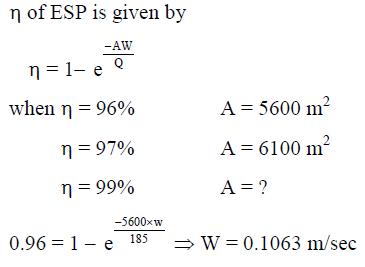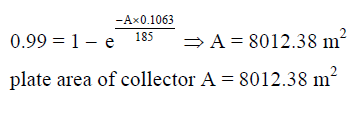*Answer can only contain numeric values
Practice Test: Gate Civil Engineering(CE) 2016 Paper - Question 62

The 2-day and 4-day BOD values of a sewage sample are 100 mg/L and 155 mg/L, respectively. The value of BOD rate constant (expressed in per day) is _________

Detailed Solution for Practice Test: Gate Civil Engineering(CE) 2016 Paper - Question 62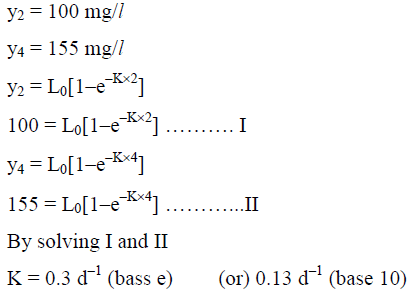Practice Test: Gate Civil Engineering(CE) 2016 Paper - Question 63

A two lane, one-way road with radius of 50 m is predominantly carrying lorries with wheelbase of 5 m. The speed of lorries is restricted to be between 60 kmph and 80 kmph. The mechanical widening and psychological widening required at 60 kmph are designated as wme,60 and wps,60, respectively. The mechanical widening and psychological widening required at 80 kmph are designated as wme,80 and wps,80, respectively. The correct values of wme,60, wps ,60, wme,80, wps,80, respectively are

Detailed Solution for Practice Test: Gate Civil Engineering(CE) 2016 Paper - Question 63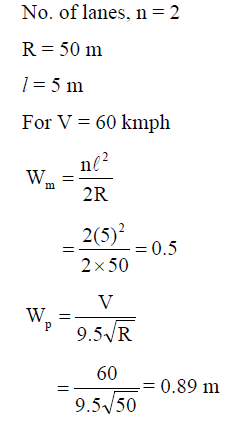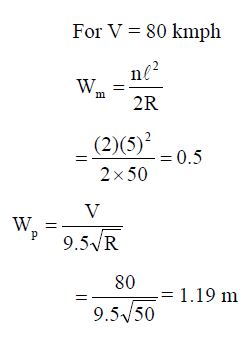*Answer can only contain numeric values
Practice Test: Gate Civil Engineering(CE) 2016 Paper - Question 64

While traveling along and against the traffic stream, a moving observer measured the relative flows as 50 vehicles/hr and 200 vehicles/hr, respectively. The average speeds of the moving observer while traveling along and against the stream are 20 km/hr and 30 km/hr, respectively. The density of the traffic stream (expressed in vehicles/km) is _________

Detailed Solution for Practice Test: Gate Civil Engineering(CE) 2016 Paper - Question 64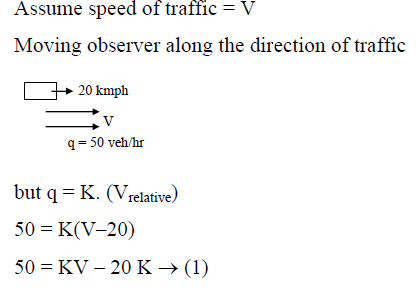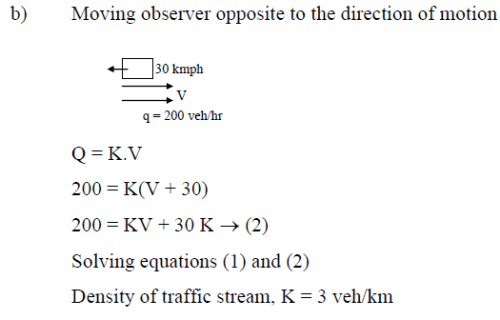*Answer can only contain numeric values
Practice Test: Gate Civil Engineering(CE) 2016 Paper - Question 65

The vertical angles subtended by the top of a tower T at two instrument stations set up at P and Q, are shown in the figure. The two stations are in line with the tower and spaced at a distance of 60 m. Readings taken from these two stations on a leveling staff placed at the benchmark (BM = 450.000 m) are also shown in the figure. The reduced level of the top of the tower T (expressed in m) is _________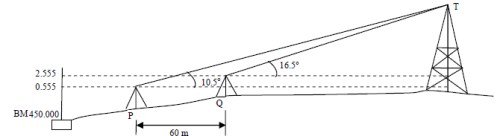Detailed Solution for Practice Test: Gate Civil Engineering(CE) 2016 Paper - Question 65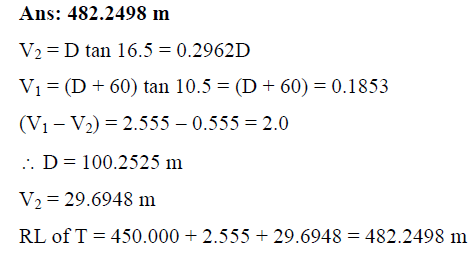Information about Practice Test: Gate Civil Engineering(CE) 2016 Paper Page
In this test you can find the Exam questions for Practice Test: Gate Civil Engineering(CE) 2016 Paper solved & explained in the simplest way possible. Besides giving Questions and answers for Practice Test: Gate Civil Engineering(CE) 2016 Paper, EduRev gives you an ample number of Online tests for practice(Scan QR code)